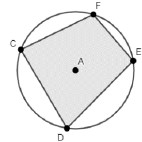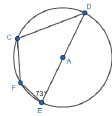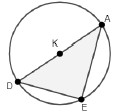# MA.912.GR.6.3Export Print
Solve mathematical problems involving triangles and quadrilaterals inscribed in a circle.

### Clarifications

Clarification 1: Instruction includes cases in which a triangle inscribed in a circle has a side that is the diameter.
General Information
Subject Area: Mathematics (B.E.S.T.)
Strand: Geometric Reasoning
Status: State Board Approved

## Benchmark Instructional Guide

### Terms from the K-12 Glossary

• Circle
• Circumscribed Circle
• Diameter
• Inscribed Polygon in a Circle
• Triangle

### Vertical Alignment

Previous Benchmarks

Next Benchmarks

### Purpose and Instructional Strategies

In grade 8, students learned about regular polygons and their interior angles. In Algebra 1, students rearranged formulas to highlight a quantity of interest and solved linear equations in one variable. In Geometry, students learn about the properties of the interior angles of triangles and quadrilaterals that are inscribed in a circle and how they relate to one another.
• Instruction includes using precise definitions and language when working with triangles and quadrilaterals inscribed in a circle. Students should be able to determine the similarities and differences between each and how their relationships interact with one another. (MTR.4.1
• For example, students should be able to answer questions like “What is the difference between a polygon inscribed in a circle and a polygon circumscribed about a circle?” and “What is the difference between a circle inscribed in a polygon and a polygon inscribed in a circle?”
• Instruction includes the connection to constructing equilateral triangles and squares inscribed in a circle.
• Instruction includes exploring various triangles inscribed in a circle.
• For example, when one side of a triangle inscribed in a circle is the diameter of the circle, then the triangle is inscribed in a semicircle. Students should determine what kind of angles are the interior angles of the triangle inscribed in a semicircle and should be able to identify them as inscribed angles. Students can make the connection to the angle opposite the diameter divides the circle into two equal parts, therefore the inscribed angle is one-half of 180°. This conclusion can lead students to Thales’ Theorem which states that the angle inscribed in semicircle is always a right angle. That is, if A, B and C are distinct points on a circle where AC is a diameter, the angle ABC is a right angle. Therefore, the triangle ABC is always a right triangle.
• Instruction includes the connection to the relationships between inscribed angles and their intercepted arcs, when a triangle is inscribed in a circle, and the Triangle Sum Theorem. When a triangle is inscribed in a circle, the three arcs intercepted by the interior angles complete a circle, so the sum of the measures of the interior angles of the triangle is half the measure of a circle in degrees, 180 °. (MTR.5.1
• A quadrilateral inscribed in a circle is also called a cyclic quadrilateral. The opposite angles of such quadrilateral are supplementary, and each exterior angle is equal to the interior opposite angle. To prove the first of these properties, students can determine what type of angles are the interior angles of the quadrilateral inscribed in a circle. Then students can discover that opposite angles of the quadrilateral have intercepted arcs such that they complete a circle. Using the relationship between inscribed angles and their intercepted arcs, students should be able to conclude that the opposite angles of cyclic quadrilaterals are supplementary.
• Instruction includes the understanding that there are two definitions of an inscribed circle in a polygon. One definition states that the inscribed circle is the largest circle that fits inside the polygon. Under this definition, all polygons have inscribed circles, with some polygons (e.g., rectangles) having more than one inscribed circle. The other definition states that the inscribed circle is the circle that fits inside of the polygon and touches all of its sides. Under this definition, only special polygons (e.g., regular polygons) have inscribed circle. Students can explore the differences by constructing an inscribed circle in a quadrilateral.
• For example, given a quadrilateral that is not a rectangle, students can extend two opposite sides to form a triangle and construct the inscribed circle of that triangle. Students can repeat this for the other two opposite sides of the quadrilateral. Students should be able to reason that one of the two constructed circles is the largest circle that fits inside the given quadrilateral.
• Extension of this benchmark includes the introduction of Ptolemy’s Theorem with cyclic quadrilaterals. This theorem states that the product of the diagonals is equal to the sum of the product of its two pairs of opposite sides. Students should realize that when this theorem is applied to a rectangle inscribed in a circle, it can be used to prove the Pythagorean Theorem.
• For example, given rectangle ABCD inscribed in circle O. Students can apply Ptolemy’s Theorem, so that ACBD = AB CD + ADBC. Since the opposite sides of a rectangle are congruent and the diagonals of a rectangle are congruent, then AB = CD, AD = BC and AC = BD. Using the Substitution Property of Equality, then AC AC = AB AB + BC BC. That is AC2 = AB2 + BC2.### Common Misconceptions or Errors

• Students may think that every quadrilateral can be inscribed in a circle. To help address this misconception, have students use a triangle inscribed in a circle to see that there are many places a fourth vertex can be placed to form a quadrilateral that cannot be inscribed in the circle.

• Quadrilateral DCFE is inscribed in Circle A and the measure of angle D is 75°.• Part A. What is the measure of angle CAE? What is the measure of angle CFE
• Part B. What is the measure of arc CDE
• Part C. What can you determine about the measures of angle DCF and angle FED?

### Instructional Items

Instructional Item 1
• In circle A, segment DE is a diameter.• Part A. Determine the measure of angle C
• Part B. If the measure of arc CF is 50°, determine the measures of angle D and angle F

Instructional Item 2
• Triangle DAE is inscribed in Circle K• Part A. Determine the value of $x$ if the measure of angle E is (2$x$ + 30)°.
• Part B. Determine the measure of angle D if the measure of angle A is (2$x$ − 20)°.

*The strategies, tasks and items included in the B1G-M are examples and should not be considered comprehensive.

## Related Courses

This benchmark is part of these courses.
1200400: Foundational Skills in Mathematics 9-12 (Specifically in versions: 2014 - 2015, 2015 - 2022, 2022 and beyond (current))
1206310: Geometry (Specifically in versions: 2014 - 2015, 2015 - 2022, 2022 and beyond (current))
1206320: Geometry Honors (Specifically in versions: 2014 - 2015, 2015 - 2022, 2022 and beyond (current))
1206315: Geometry for Credit Recovery (Specifically in versions: 2014 - 2015, 2015 - 2022, 2022 and beyond (current))
7912065: Access Geometry (Specifically in versions: 2015 - 2022, 2022 and beyond (current))

## Related Access Points

Alternate version of this benchmark for students with significant cognitive disabilities.
MA.912.GR.6.AP.3: Identify and describe the relationship involving triangles and quadrilaterals inscribed in a circle.

## Related Resources

Vetted resources educators can use to teach the concepts and skills in this benchmark.

## Formative Assessments

Students are asked to prove that opposite angles of a quadrilateral, inscribed in a circle, are supplementary.

Type: Formative Assessment

The Sprinters’ Race:

Students are given a grid with three points (vertices of a right triangle) representing the starting locations of three sprinters in a race and are asked to determine the center of the finish circle, which is equidistant from each sprinter.

Type: Formative Assessment

## MFAS Formative Assessments

Students are asked to prove that opposite angles of a quadrilateral, inscribed in a circle, are supplementary.

The Sprinters’ Race:

Students are given a grid with three points (vertices of a right triangle) representing the starting locations of three sprinters in a race and are asked to determine the center of the finish circle, which is equidistant from each sprinter.

## Student Resources

Vetted resources students can use to learn the concepts and skills in this benchmark.

## Parent Resources

Vetted resources caregivers can use to help students learn the concepts and skills in this benchmark.